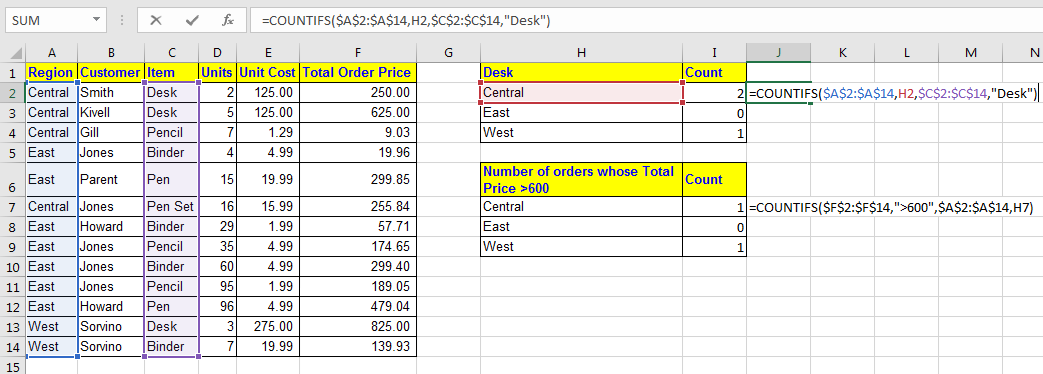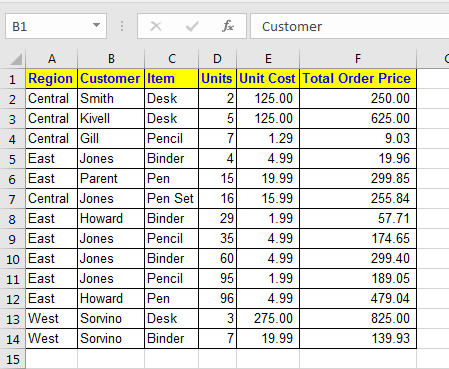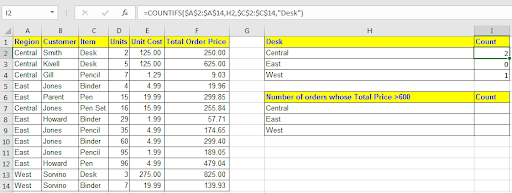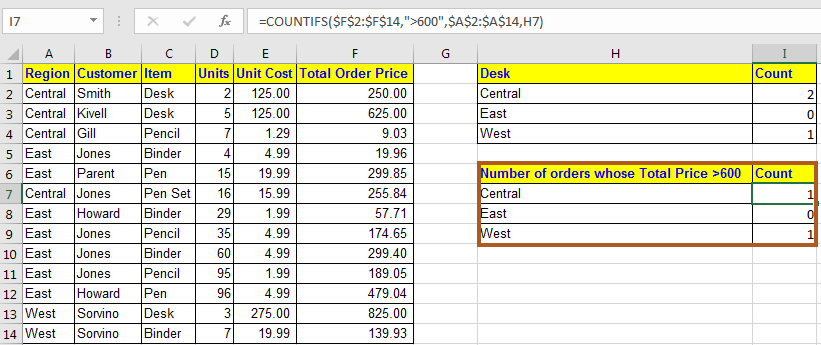# How to use the COUNTIFS Function n ExcelWe learned about COUNTIF in Excel to count values on one condition. It gets tricky when you want to count on multiple conditions.

Excel provides COUNTIFS function that counts on multiple conditions.

=COUNTIF(range1, criteria1, [range2], [criteria2],...)

Range1 : first range for criteria1.
Criteria1 : criteria from range1.
Range2 : range for criteria2. Optional.
Criteria1 : criteria from range2. Optional.

...

Lets see Excel’s COUNTIFS function in action:
Scenario:
Assume you have this data of Stationary Orders:Now you have to prepare these reports:In first query, we need to count orders of Desk from Central, East and West.
First criteria is Desk from Item column.
Second criteria is region in cell H2, H3 and H4 (Central, East and West whichever).
Write this COUNTIFS formula in I2 and drag it down.

=COUNTIFS(\$A\$2:\$A\$14,H2,\$C\$2:\$C\$14,"Desk")

And walla! COUNTIFS in Excel counted on the number of orders of desk in each Region. Instead of “Desk”, you can give reference of cell H1 as we did for Region, so that it will show number of any item written in H1.Next we need to find number of orders for each region whose order price is greater than 600

First condition is >600 from Total Order Price.
Second criteria is region in cell H2, H3 and H4 (Central, East and West whichever).

Write this COUNTIFS formula in cell I7 and drag it down.

=COUNTIFS(\$F\$2:\$F\$14,">600",\$A\$2:\$A\$14,H7)

You will see the counts of given conditions.Pro Notes:

• With numarice criterias you don’t need to use double quotes. However while working with logical operators (<,>,=) you need to use double quotes.
• Each range should have same number of columns and rows.
• Wild card characters(*,?) are allowed in COUNTIFS function.

Here I discussed briefly about COUNTIFS function in Excel. It is one most powerful function in excel and has many uses. If you have any counting problem in excel and you are stuck, comment down below. We will tear down that problem together.

Related Articles:

How to Use REPLACE function in Excel

How to Use SUBSTITUTE function in Excel

How to use the Excel SEARCH function

Popular Articles:

50 Excel Shortcuts to Increase Your Productivity

How to use the VLOOKUP Function in Excel

How to use the COUNTIF function in Excel 2016

How to Use SUMIF Function in Excel

Terms and Conditions of use

The applications/code on this site are distributed as is and without warranties or liability. In no event shall the owner of the copyrights, or the authors of the applications/code be liable for any loss of profit, any problems or any damage resulting from the use or evaluation of the applications/code.